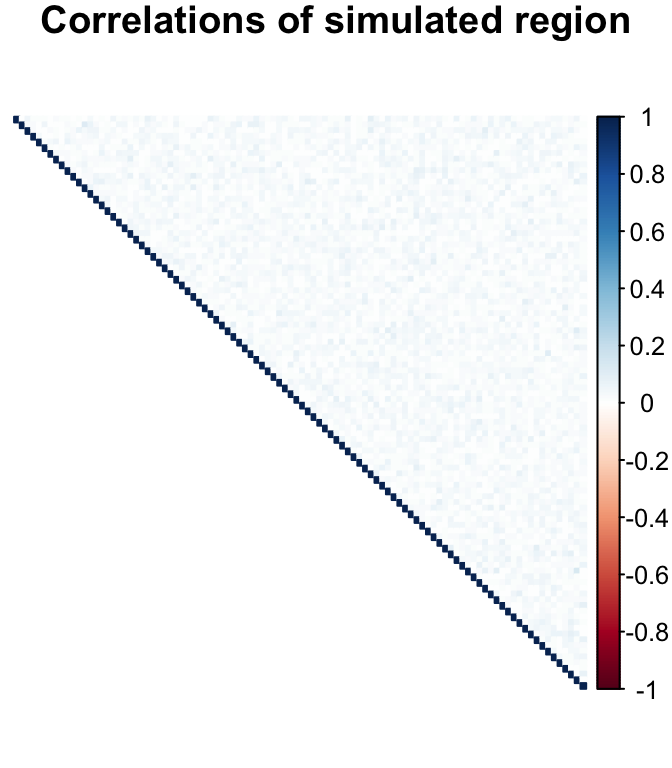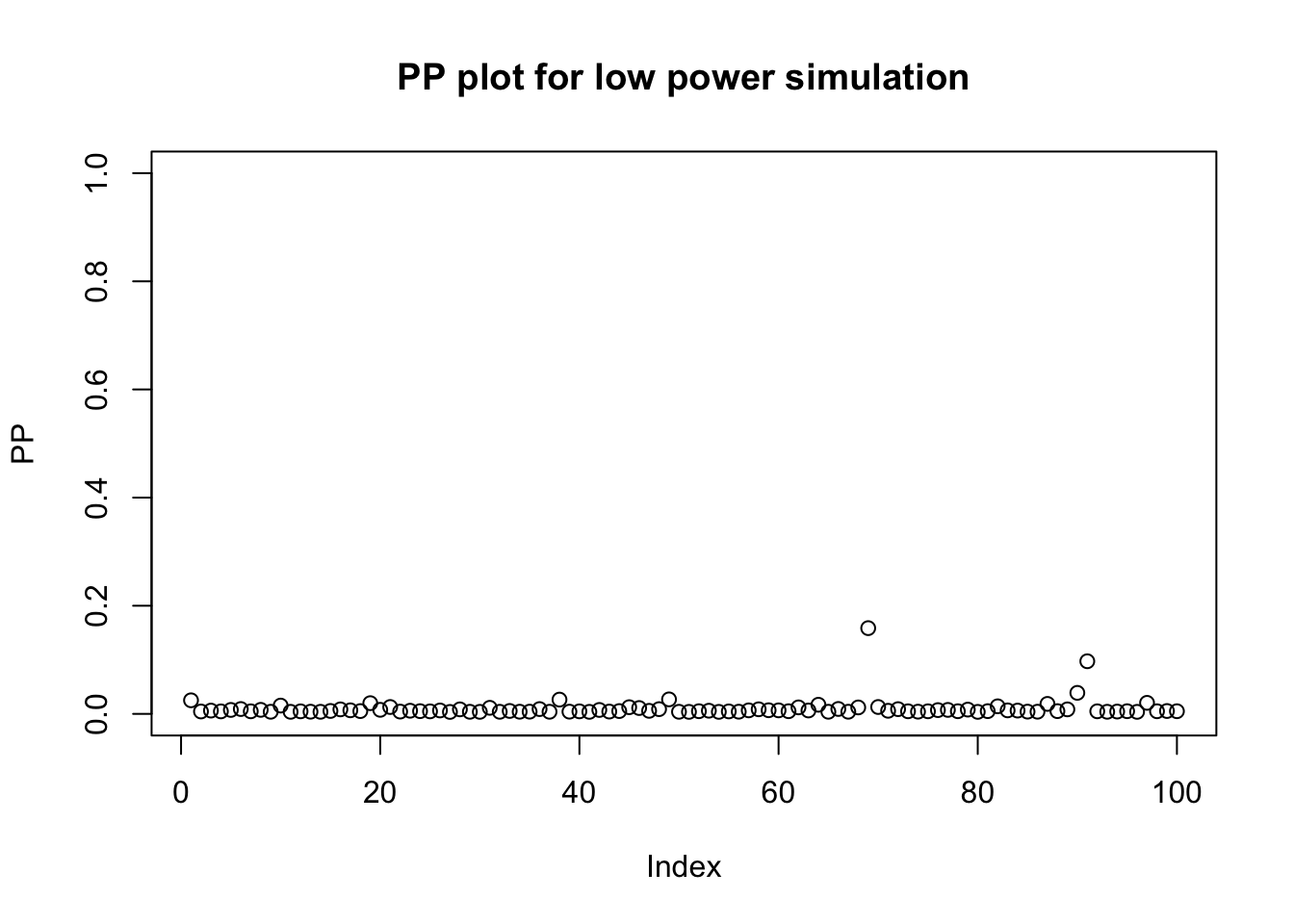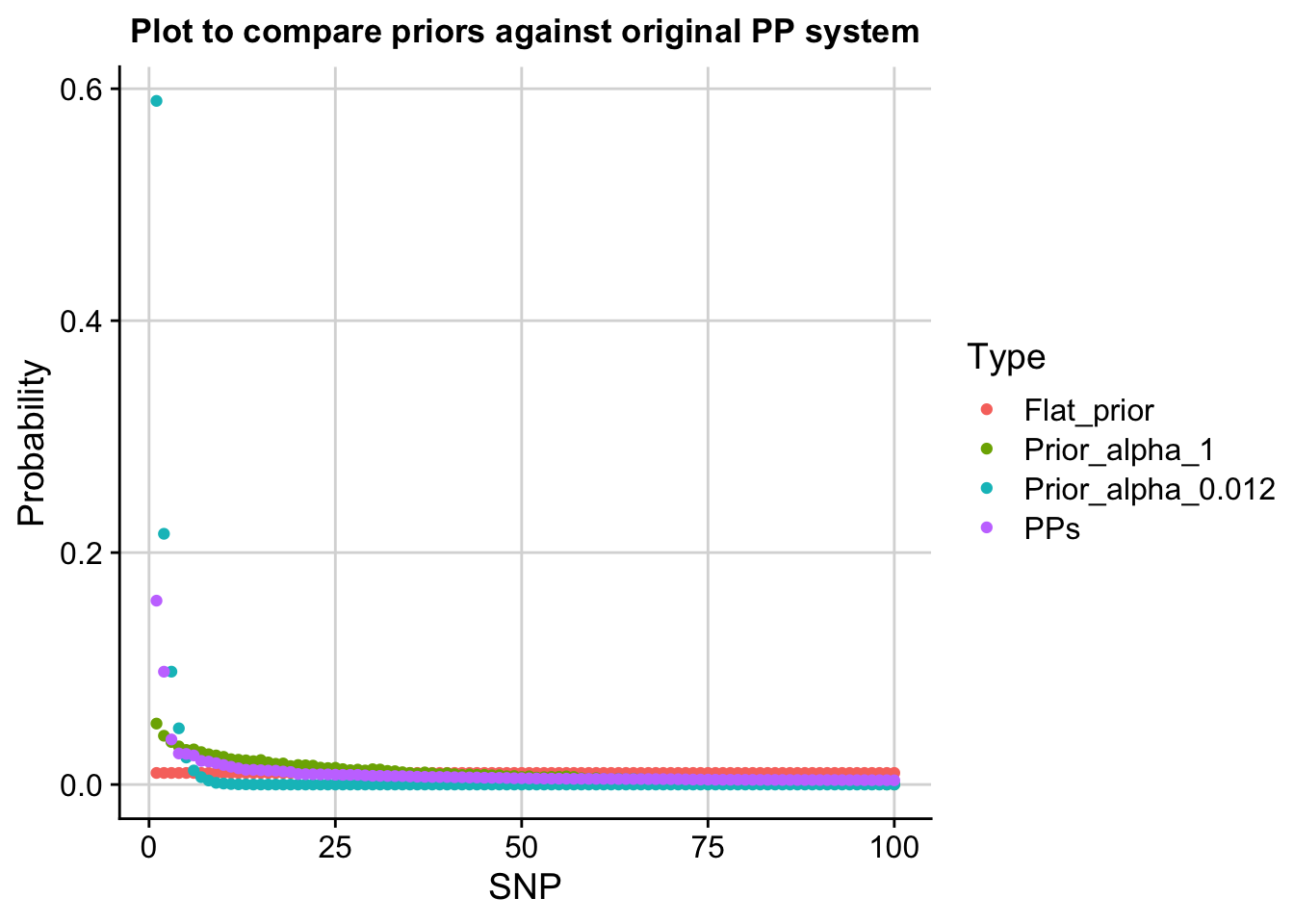Note: Code for all of this is on HPC in reweight_prior/new_alpha

### Introduction

In the Dirichlet distribution, the concentration parameters, $$\boldsymbol{\alpha}=(\alpha_1, ..., \alpha_n)$$, control the shape of the distibrution. In our method we assume that these parameters are all equal so that $$\alpha_i=\alpha \text{ for } i=1,...,n$$. The sum of these, $$\alpha_0=\sum\alpha_i=n\times \alpha$$, controls the strength of the distirbution (i.e. how peaked it is). If $$\alpha<1$$ there are spikes at the corners of the simplex and if $$\alpha>1$$, the distribution tends to the middle of the simplex (gets flatter). As $$\alpha_0$$ increases, the distribution becomes more tightly concentrated around the centre of the simplex.

We’ve identified that the value for $$\alpha$$ needs to vary across studies so that the shape of the reweighted prior accurately reflects our prior belief of variant causality. That is, in lower powered scenarios the resultant reweighted prior should be flatter and in higher powered scenarios it should be steeper. This reflects the belief that in lower powered scenarios we do not believe that the ordering of SNPs tells us much about variant causality whilst in higher powered scenarios we expect that the ordering is indicative of variant causality.

Herein, I consider two approaches to estimate the parameter of the Dirichlet distribution, $$\alpha$$, for a simulated genomic region with no correlations between SNPs:

1. $$\alpha = \frac{1}{sum(BF)}$$

2. Use the entropy of the system to estimate $$\alpha$$.

The idea is that if we can find a method that works in the instance of no SNP correlations, then we can extend it to situations where there are SNP correlations.### 1. Set $$\alpha = \frac{1}{sum(BF)}$$

I use alpha=1/exp(logsum(labf)).

#### Low power

In low power, we’ve already seen that using $$\alpha=1$$ upweights the top variant too much and we become “too sure” that this variant is the causal variant. We hope that using this new method to select $$\alpha$$ will increase the value of alpha, therefore making the prior flatter.

Low power –> exp(denom) low –> $$\alpha$$ big –> flatter prior.

Let’s consider an example.

# low power simulation
NN = 1000
OR = 1.05

tmp <- simref(N0 = NN, N1 = NN, OR = OR)
maf <- tmp$maf varbeta <- Var.data.cc(maf, N=2*NN, 0.5) # variance of beta z_low <- tmp$z
pp_low <- ppfunc(z_low, varbeta)

plot(pp_low, ylim = c(0,1), ylab = "PP", main = "PP plot for low power simulation")mu <- est_mu(z = z_low, f = maf, N0 = NN, N1 = NN)
mu
##  1.581177
o <- order(pp_low, decreasing = TRUE)

W = 0.2
V = varbeta
r = W^2/(W^2 + V)
lbf = 0.5 * (log(1 - r) + (r * z_low^2))

abf <- exp(lbf)
# alpha_1 = 1/sum(abf)
# alpha_2 = 1/sum(lbf)
# alpha_3 = 1/logsum(lbf)

# data.frame(alpha_1, alpha_2, alpha_3)

denom = logsum(lbf)
alpha = 1/exp(denom)

nsnps = length(maf)

prior <- rowMeans(sapply(1:50000, function(i) draw_order(nsnps, alpha)))

o_w = order(abf, decreasing=TRUE)
ordered_pp = abf[o_w]*prior/sum(abf[o_w]*prior)

# for comparison do alpha = 1

alpha_1 = 1

prior_1 <- rowMeans(sapply(1:50000, function(i) draw_order(nsnps, alpha_1)))

ordered_pp_1 = abf[o_w]*prior_1/sum(abf[o_w]*prior_1)Above, the purple points show the PPs calculated using the standard method, the red points show the flat prior, the green points show the prior where $$\alpha=1$$ and the blue points where $$\alpha=\frac{1}{sum(BF)}$$.

We had hoped that using this method would select a value for $$\alpha$$ that is $$>>1$$, instead we find it selects a tiny value for $$\alpha$$ therefore making the prior way too steep.